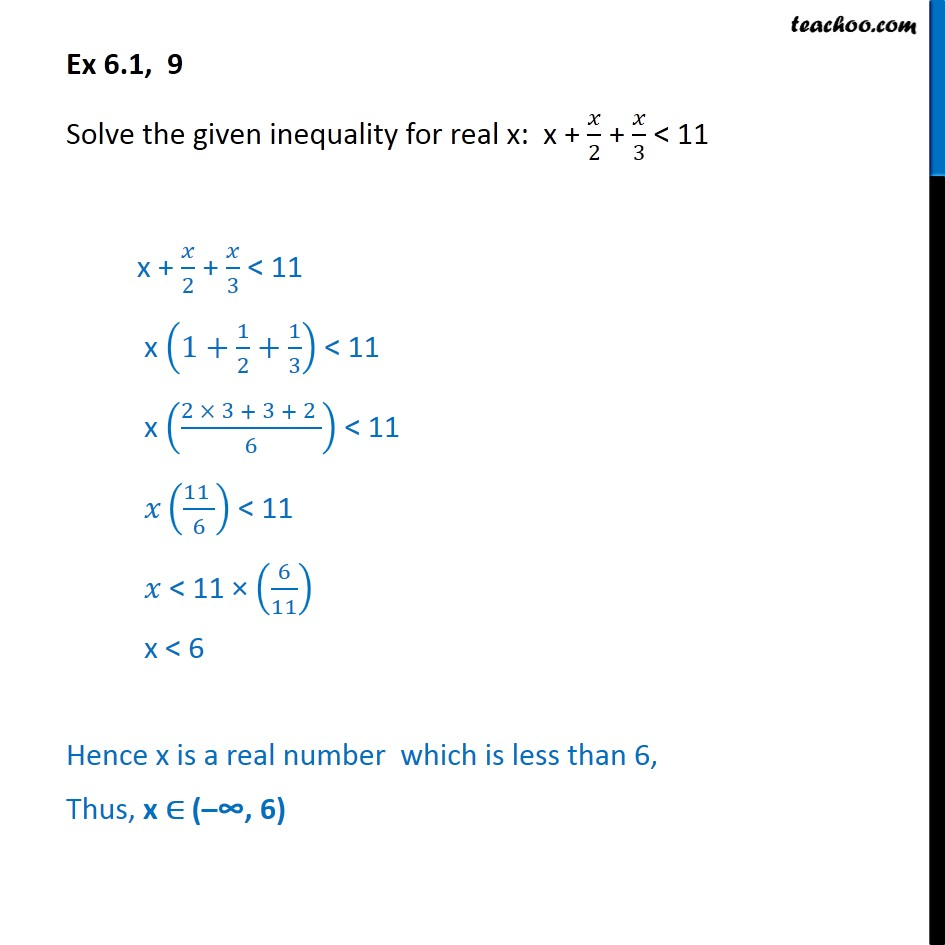1. Chapter 6 Class 11 Linear Inequalities (Term 2)
2. Serial order wise
3. Ex 6.1

Transcript

Ex 6.1, 9 Solve the given inequality for real x: x + 2 + 3 < 11 x + 2 + 3 < 11 x 1+ 1 2 + 1 3 < 11 x 2 3 + 3 + 2 6 < 11 11 6 < 11 < 11 6 11 x < 6 Hence x is a real number which is less than 6, Thus, x ( 6)

Ex 6.1